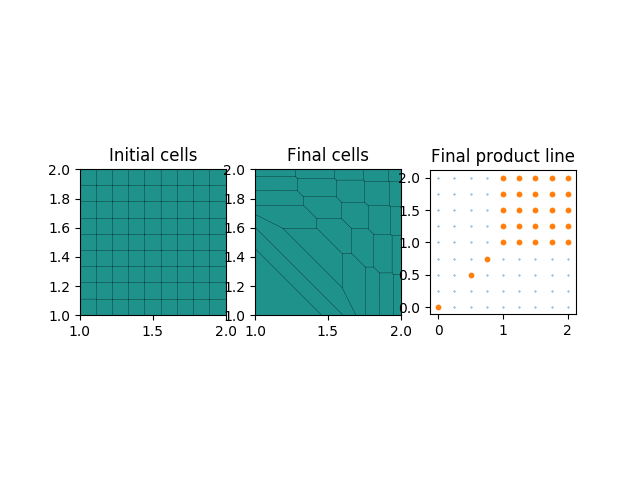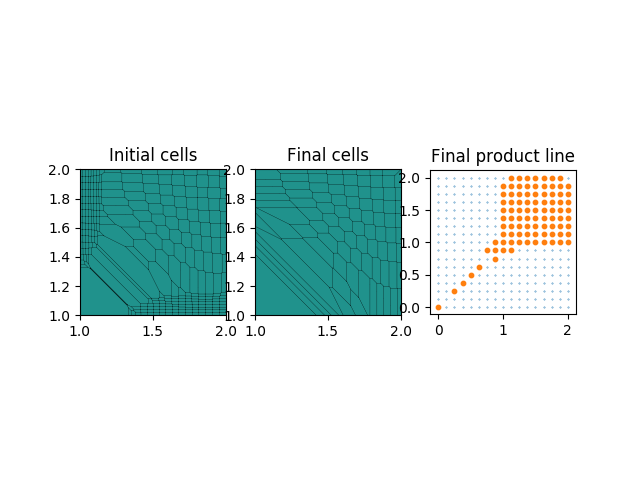# Semi-discrete principal-agent problem¶

###### Jean-David Benamou, Xavier Dupuis¶

using PyMongeAmpere by Quentin Mérigot: https://github.com/mrgt/PyMongeAmpere.

## Setting¶

We consider the monopolist's problem in a semi-discrete setting: given

• a set of agents' types $X \subset \mathbb R^d$ convex and bounded;
• a distribution of agents' types $\mu \in \mathcal P(X)$ absolutely continuous;
• a set of products' types $Y = \{ y_0, \ldots, y_N \} \subset \mathbb R^d$ with $y_0$ an outside option;
• a vector of associated costs $(c_0, \ldots, c_N) \in \mathbb R^{N+1}$;

the monopolist is looking for

• a price schedule $v=(v_1, \ldots, v_N) \in \mathbb R^N$ ($v_0=c_0$ is fixed for the outside option) that maximizes her profits, i.e. that solves (SDPAP):
$$\min_{v \in \mathbb R^N} \sum_{i=1}^N (c_i -v_i)\ \mu\left( \text{Lag}_i(v) \right)$$

where the cell $\text{Lag}_i(v) := \left\{ x \in X : b(x,y_i)-v_i \ge b(x,y_j)- v_j, \ \forall j=0,\ldots,N \right\}$ is the set of agents' types $x$ that choose, in response to the price schedule $v$, to buy a product of type $y_i$ at the price $v_i$ (because it maximizes their quasi-linear utility).

We restrict in what follows to the bilinear valuation function $b(x,y) = x \cdot y$, so that $\left(\text{Lag}_i(v)\right)_i$ are the cells of a Laguerre-Voronoi or power diagram. We further restrict to the dimension $d=2$.

## Algorithm¶

Let

• $L = (\frac{1}{N+1}, \ldots, \frac{1}{N+1}) \in \mathbb R^{N+1}$;

for $k = 1, \ldots, \text{multi}$, let

• $v \in \mathbb R^N$ be such that $\left( \mu\left( \text{Lag}_i(v) \right) \right)_i = L$ (obtained by solving a semi-discrete optimal transport problem, here in dimension 2 with PyMongeAmpere by Quentin Mérigot);
• $\tilde v \in \mathbb R^N$ be obtained by numerical minimization of the objective function, with initial condition $v$ (here the L-BFGS-B algorithm of SciPy);
• $\tilde L = \left( \mu\left( \text{Lag}_i(\tilde v) \right) \right)_i \in \mathbb R^{N+1}$ (computed here from PyMongeAmpere by Quentin Mérigot);
• $L = \frac{1}{2} (L + \tilde L)\in \mathbb R^{N+1}$;

return $(\tilde v, \tilde L)$.

### Uniform distribution on a square¶

$X=[1,2]^2$, $\mu$ uniform, $Y$ a regular grid of $[0,2]^2$, $c(y) =\frac{1}{2} |y|^2$

Run (shift+enter) the cell below. One can choose $N$ via the argument $n$ ($N = (2^n+1)^2$) and the argument multi.

In :
% matplotlib notebook
% run QuadUnifSquare.py --n 3 --multi 1
# n -> (2^n+1)^2 products' types, multi -> number of successive optimizations

DATA
Number of products' types: 81, distribution of agents' types: uniform on a square, cost: quadratic
OPTIMIZATION 1
Initial function value: -1.324290
Optimization method: L-BFGS-B, status: 0, success: True, nit: 61, CONVERGENCE: REL_REDUCTION_OF_F_<=_FACTR*EPSMCH
Final function value: -1.508670In :
% matplotlib notebook
% run QuadUnifSquare.py  --n 4 --multi 3
# n -> (2^n+1)^2 products' types, multi -> number of successive optimizations

DATA
Number of products' types: 289, distribution of agents' types: uniform on a square, cost: quadratic
OPTIMIZATION 1
Initial function value: -1.330908
Optimization method: L-BFGS-B, status: 0, success: True, nit: 223, CONVERGENCE: REL_REDUCTION_OF_F_<=_FACTR*EPSMCH
Final function value: -1.513311
OPTIMIZATION 2
Initial function value: -1.439223
Optimization method: L-BFGS-B, status: 0, success: True, nit: 176, CONVERGENCE: REL_REDUCTION_OF_F_<=_FACTR*EPSMCH
Final function value: -1.512956
OPTIMIZATION 3
Initial function value: -1.478573
Optimization method: L-BFGS-B, status: 0, success: True, nit: 189, CONVERGENCE: REL_REDUCTION_OF_F_<=_FACTR*EPSMCH
Final function value: -1.513380In [ ]:
% matplotlib notebook
% run QuadUnifSquare.py --n 5 --multi 3
# n -> (2^n+1)^2 products' types, multi -> number of successive optimizations


### Gaussian distribution on a square¶

$X=[1,2]^2$, $\mu$ Gaussian, $Y$ a regular grid of $[0,2]^2$, $c(y) =\frac{1}{2} |y|^2$

Run (shift+enter) the cell below. One can choose $N$ via the argument $n$ ($N = (2^n+1)^2$), the standard deviation $\sigma$ of $\mu$, and the argument multi.

In [ ]:
% matplotlib notebook
% run QuadGaussianSquare.py --n 3 --sigma 5 --multi 3
# n -> (2^n+1)^2 products' types, sigma -> standard deviation, multi -> number of successive optimizations


### Gaussian distribution on a disk¶

$X$ the disk inscribed in $[1,2]^2$, $\mu$ Gaussian, $Y$ a regular grid of $[0,2]^2$, $c(y) =\frac{1}{2} |y|^2$

Run (shift+enter) the cell below. One can choose $N$ via the argument $n$ ($N = (2^n+1)^2$), the standard deviation $\sigma$ of $\mu$, and the argument multi.

In [ ]:
% matplotlib notebook
% run QuadGaussianDisk.py --n 4 --sigma 10 --multi 3
# n -> (2^n+1)^2 products' types, sigma -> standard deviation, multi -> number of successive optimizations


## Chinese SDPAP¶

### Uniform distribution on a square¶

$X=[0,1]^2$, $\mu$ uniform, $Y$ a regular grid of $[0,1]^2$, $c(y) \equiv 0$

Run (shift+enter) the cell below. One can choose $N$ via the argument $n$ ($N = (2^n+1)^2$) and the argument multi.

In [ ]:
% matplotlib notebook
% run ChineseUnifSquare.py --n 5 --multi 1
# n -> (2^n+1)^2 products' types, multi -> number of successive optimizations

In [ ]:
% matplotlib notebook
% run ChineseUnifSquare.py --n 3 --multi 3
# n -> (2^n+1)^2 products' types, multi -> number of successive optimizations